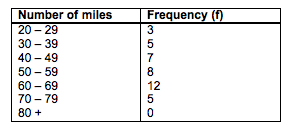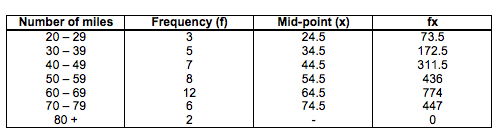Statistics and Probability

Grouped data

## Measurements using grouped or continuous dataIf there’s a lot of data to deal with it can be easier to group the data into classes. As an example for this, cyclists at a cycling club were asked how far they cycled on average each week:

Mean

Finding the mean from a frequency table is very similar to finding it from grouped or continuous data.

At the moment we don’t have exact figures to work with. So, first of all you need to calculate the mid-points of, in this example, the number of miles. You then multiply this mid point by the frequency:The mean is then the sum of fx divided by the sum of f:

Sum of fx = 73.5 + 172.5 + 311.5 + 436 + 774 + 447 = 2214.5

Sum of f = 3 + 5 + 7 + 8 + 12 + 6 + 2 = 43

mean = 2214.5 43= 51.5

Remember that because there are no exact figures to work with this mean is only an estimate.

#### The medianAs you may remember the median refers to the middle number once all the results have been put in numerical order.

Again, we can only find an estimate rather than an exact value.

There are 43 cyclists so to find the nth value:

(43 + 1) 2 = 22nd value

Therefore the median is in the class 50 – 59.

#### The mode

The mode is the result that appears the most.

We can’t find a mode but we can see what is the modal class which, in this example, is 60 – 69.